Ch 10. Vibrations Multimedia Engineering Dynamics Free Vibs. Undamped Free Vibs. Damped Forced Vibration Energy Method
 Chapter - Particle - 1. General Motion 2. Force & Accel. 3. Energy 4. Momentum - Rigid Body - 5. General Motion 6. Force & Accel. 7. Energy 8. Momentum 9. 3-D Motion 10. Vibrations Appendix Basic Math Units Basic Equations Sections Search eBooks Dynamics Fluids Math Mechanics Statics Thermodynamics Author(s): Kurt Gramoll ©Kurt GramollDYNAMICS - CASE STUDY SOLUTION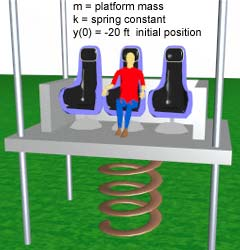Problem Parameters A free-body diagram can be used to identify all the forces on a the platform. The weight, mg, will act on the spring but the spring force will counter-act the weight, so the weight actually cancels. The spring deflection will cause a force, ky, that will accelerate the platform. The final equation of motion becomes,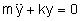The general solution of this differential equation is       y(t) = A sinωnt + B cosωnt where ωn represents the natural frequency and is defined as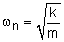For the two cases, k1 = 5,000 and k2 = 10,000, the natural frequencies are found:      case 1:   ωn1 = 2.24 rad/s      case 2:   ωn2 = 3.16 rad/s The initial conditions for this particular situation are      y(0) = -20 ft      dy(0)/dt = 0 These two conditions can be used to determined the constants A and B. The final equation becomes      y(t) = -20 cosωnt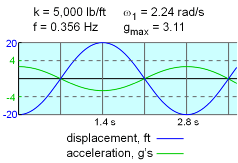Case 1 Results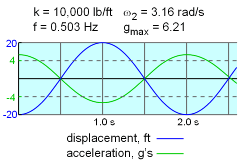Case 1 Results The velocity equation is determined by taking the time derivative of the displacement equation,      dy/dt = -20 ωn sinωnt Likewise, the acceleration equation is found taking the second time derivative of the displacement,      d2y/dt2 = -20 ωn2 cosωnt Therefore, the maximum acceleration is directly related to the square of the natural frequency, or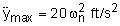This can be converted to g forces by dividing by the acceleration of gravity,      gmax = 20/32.2 ωn2 = 0.621ωn2 g's The motion of the platform and its acceleration are plotted at the left for the two spring constants of 5,000 and 10,000 lb/ft. The 10,000 lb/ft case is unacceptable since the acceleration exceeds the safety limit of 4 g's.

Practice Homework and Test problems now available in the 'Eng Dynamics' mobile app
Includes over 400 problems with complete detailed solutions.
Available now at the Google Play Store and Apple App Store.